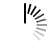# Parity Check of a Number

Parity of a number is based on the number of 1’s present in the binary equivalent of that number. When the count of present 1s is odd, it returns odd parity, for an even number of 1s it returns even parity.

As we know that the numbers in computer memory are stored in binary numbers, so we can shift numbers easily. In this case, by shifting the bits, we will count a number of 1’s present in the binary equivalent of the given number.

#### Input and Output

```Input:
A number: 5
Binary equivalent is (101)
Output:
Parity of 5 is Odd.```

#### Algorithm

`finParity(n)`

Input: The number n.

Output: Check the number has even parity or odd parity.

```Begin
count := 0
temp := n

while temp >= 2, do
if temp has 1 as LSb, then
count := count + 1
temp := right shift temp for 1 bit
done

if count is odd number, then
display it is odd parity
else
display even parity
End```

#### Source Code (C++)

```#include <iostream>
using namespace std;

bool findParity(int n) {
int count = 0;
int temp = n;

while (temp>=2) {
if(temp & 1)    //when LSb is 1, increase count
count++;
temp = temp >> 1;    //right shift number by 1 bit
}
return (count % 2)?true:false;
}

int main() {
int n;
cout << "Enter a number: "; cin >>n;
cout << "Parity of " << n << " is " << (findParity(n)?"Odd":"Even");
}```

#### Output

```Enter a number: 5
Parity of 5 is Odd```